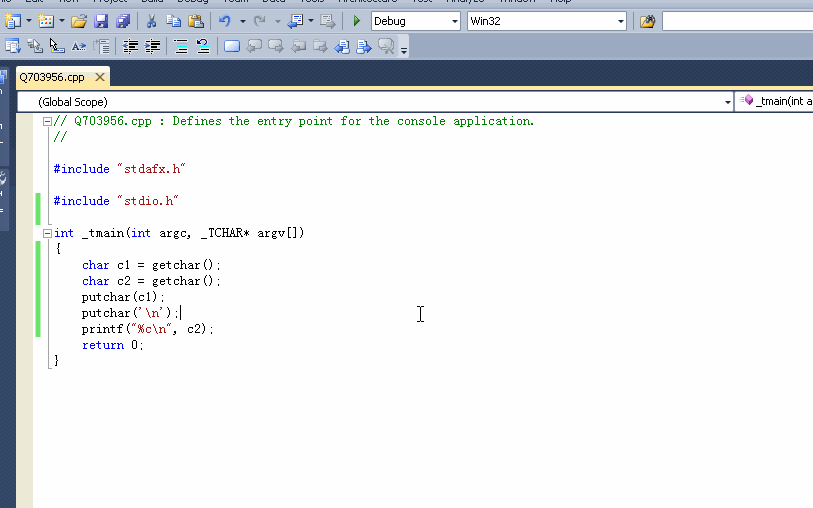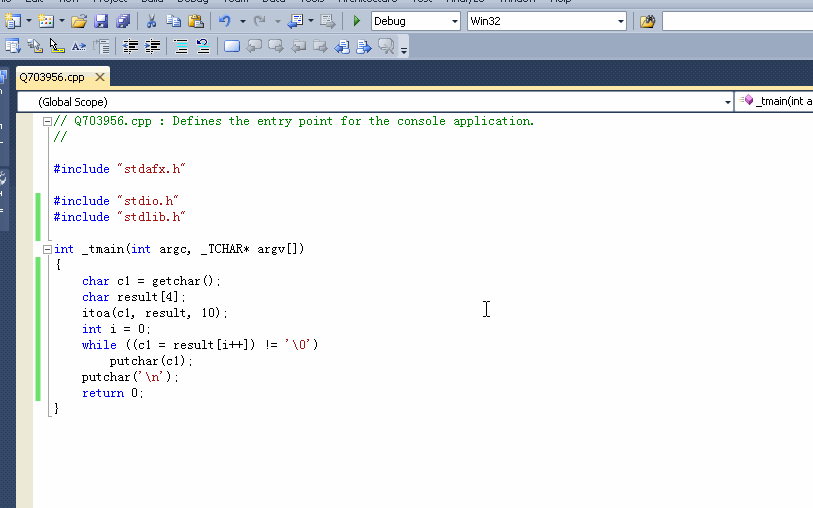2）要求输出c1和c2的ASCII码，用putchar还是printf函数？
3）整形变量和字符型变量是否可在任何情况下互相替代？

2个回答

``````#include "stdio.h"

int _tmain(int argc, _TCHAR* argv[])
{
char c1 = getchar();
char c2 = getchar();
putchar(c1);
putchar('\n');
printf("%c\n", c2);
return 0;
}
``````1）变量c1和c2应定义为字符型还是整形变量，还是二者皆可？

2）要求输出c1和c2的ASCII码，用putchar还是printf函数？

``````#include "stdio.h"
#include "stdlib.h"

int _tmain(int argc, _TCHAR* argv[])
{
char c1 = getchar();
char result;
itoa(c1, result, 10);
int i = 0;
while ((c1 = result[i++]) != '\0')
putchar(c1);
putchar('\n');
return 0;
}
``````3）整形变量和字符型变量是否可在任何情况下互相替代？

`采纳本回答，留下email送我的资源：C语言源代码大全源代码上百个：https://download.csdn.net/download/caozhy/9919273`

1.定义字符型存的是字符，整型的存的是字符的ASCII码，二者是不同的，如果你输出结果一样，是因为你输出语句的格式控制符，都以字符%c输出，整型会转为char输出。

2.ASCII码是一个数字，数字肯定用整型，所以用printf，且格式控制符为%d

3.可以相互转化，不理解你说的替代是什么意思getchar（）函数到底干什么用的啊？为什么字符比较要用getchar？

#include <stdio.h> void main() { int n=0; printf("input a string:\n"); while( getchar()!='\n' ) { n++; } printf("%d",n); }

C Primer Plus编程题8.2

C Prime Plus的第六版第六章第十二题怎么做？
** 这是题目要求： ** /*考虑下面两个无限序列： 1.0 + 1.0/2.0 + 1.0/3.0 + 1.0/4.0 + ... 1.0 - 1.0/2.0 + 1.0/3.0 - 1.0/4.0 + ... 编写一个程序计算这两个无限序列的总和，直到到达某次数。提示：奇 数个-1 相乘得-1，偶数个-1相乘得1。让用户交互地输入指定的次数，当用 户输入0或负值时结束输入。查看运行100项、1000项、10000项后的总和， 是否发现每个序列都收敛于某值？*/ **这是我的编程： ** #include<stdio.h> #include<math.h> int main() { int i; int num=0; double sum1=0.0; double sum2=0.0; printf("Please enter the value of the specified number of items:"); while(scanf_s("%d",&num)==1) { getchar(); for(i=1;i<=num;i++) { sum1+=(1.0/i); sum2+=pow (-1.0,i-1)*(1.0/i); } printf("1.0 + 1.0/2.0 + 1.0/3.0 + 1.0/4.0 + ...=%lf\n",sum1 ); printf("1.0 - 1.0/2.0 + 1.0/3.0 - 1.0/4.0 + ...=%lf\n",sum2 ); printf("Please enter the value of the specified number of items:"); } getchar(); getchar(); return 0; } 这是编译结果：![图片说明](https://img-ask.csdn.net/upload/201810/11/1539272974_242419.png) 编译结果是错误的。但是我完全不知道原因，诶。 如果不麻烦的话，希望有人能够给我解答一下，自学感觉有点困难。

C如何实现清空缓冲区，fflush（stdin）不行

c语言if语句the c programming language 第二版练习题
#include <stdio.h> #include <windows.h> int main() { int a,b; b = 0; while ( (a = getchar()) != EOF) { if ( a == ' ') { if (b == 0) { b =1; putchar(a); }; }; if ( a != ' ') { b = 0; putchar (a); }; }; system("pause"); return 0; } 我想问的是: if (b == 0) { b =1; putchar(a); }; 这段if语句中运行到b = 1 时这段if语句会退出吗? 此题来自the c programming language 第二版中exercise 1-9 wirte a program to copy its input to its output ,replacting each string of one or more blanks by a single blank.刚学 谢谢各位了

#include <stdio.h> int main() { int d,n=0,i=0,T; char s1={},s2={}; scanf("%d",&T); while(T--) {int b =1; char s1={},s2={}; gets(s1); gets(s2); getchar(); while(s1[n] != '\0') { n++; } d = n; while(s2[i] != '\0') { i++; } printf("Case #%d:\n",b); for(n = 0;n <= d;n++) { if(s1[n]==s2&&s1[n+i]==s2[i]) printf("%d",n); } b++; } return 0; }![图片说明](https://img-ask.csdn.net/upload/201911/30/1575126091_541451.png)

《C程序设计语言》里的一道习题，这样写为什么只能输出空格前面的部分？

C语言大三3道作业题，求大神做一下，贴代码就行！
1编写程序实现：输入任意一个正整数，判断其为几位数，输出结果。 要求：定义函数int mynum(int a),实现判断a是几位数，在main方法中输入任意正整数，调用mynum，输出结果。 2编写程序实现：输入任意一个年份，判断其是否是闰年，输出结果 要求：定义函数isleap实现判断年份是否是闰年，在main中进行输入，调用函数，输出结果。 闰年的条件是符合下面二者之一； 能被4整除，但不能100整除，如2006 能被400整除，如2000 3. 输入一行字符，统计期中的英文字母、数字、空格和其他字符个数。(30分 每空6分) #include<stdio.h> int main() { int n1,n2,n3,n4; char c; n1=n2=n3=n4=0; c=getchar(); while((1) !=‘(2)’) { if(c>=‘a’&& c<=‘z’(3) c>=‘A’&&c<=‘Z’) n1++; else if (c>=‘0’&& c<=‘9’) n2++; else if(c(4)) n3++; (5) n4++; c=getchar(); } printf(“%d %d %d %d\n”,n1,n2,n3,n4); return 0; } (1) _____________________________________ (2) _____________________________________ (3) _____________________________________ (4)_____________________________________ (5)_____________________________________
poj 2386这都题 这么做 哪里错了？ 求大神指点
``` #include<stdio.h> int n,m; char field; int dx,dy,nx,ny,res; void dfs(int x,int y ) { field[x][y]='.'; for(dx=-1;dx<=1;dx++) { for(dy=-1;dy<=1;dy++) { nx=dx+x; ny=dy+y; if(0<=nx&&nx<n&&0<=ny&&ny<m&&field[nx][ny]=='W')dfs(nx,ny); } } } int main() { int i,j; scanf("%d%d",&n,&m); for(i=0;i<n;i++) { getchar(); for(j=0;j<m;j++) { scanf("%c",&field[i][j]); } } res=0; for(i=0;i<n;i++) { for(j=0;j<m;j++) { if(field[i][j]=='W') { dfs(i,j); res++; } } } printf("%d\n",res); return 0; } ```
hdu的1558题，为何这样写代码，会memory limit exceed
``` #include<stdio.h> #include<cmath> using namespace std; struct Point { double x, y; Point(double X = 0, double Y = 0) { x = X, y = Y; } Point operator + (Point B) { return Point(x + B.x, y + B.y); } Point operator - (Point B) { return Point(x - B.x, y - B.y); } Point operator * (double k) { return Point(x*k, y*k); } Point operator / (double k) { return Point(x / k, y / k); } }; struct Line { Point p1, p2; Line() {} Line(Point P1, Point P2) { p1 = P1; p2 = P2; } }; typedef Point Vector; const double eps = 1e-8; Line line; int pre; int sgn(double a) { if (fabs(a) < eps) return 0; else return a < 0 ? -1 : 1; } double Cross(Vector A, Vector B) { return B.y*A.x - B.x*A.y; } bool Cross_segment(Point a, Point b, Point c, Point d) { //判断两线段是否相交 double c1 = Cross(b - a, c - a), c2 = Cross(b - a, d - a); double d1 = Cross(d - c, a - c), d2 = Cross(d - c, b - c); return sgn(c1)*sgn(c2) < 0 && sgn(d1)*sgn(d2) < 0; //1相交 0不相交 } int find(int u) { //并查集搜索 return pre[u] == u ? u : pre[u] = find(pre[u]); } int main() { int ccase; scanf("%d", &ccase); while (ccase--) { for (int i = 0; i < 100; i++) pre[i] = i; int k = 1; //线段数 char t; int n; scanf("%d", &n); //指令数 while (n--) { t = getchar(); while (t == ' ' || t == '\n') t = getchar(); if (t == 'P') { scanf("%lf%lf%lf%lf", &line[k].p1.x, &line[k].p1.y, &line[k].p2.x, &line[k].p2.y); k++; for (int i = 1; i < k - 1; i++) { if (Cross_segment(line[k - 1].p1, line[k - 1].p2, line[i].p1, line[i].p2)) { //如果两线段相交 int x = pre[k - 1]; int y = pre[i]; if (x != y) pre[x] = y; } } } else { int x; int num = 0; scanf("%d", &x); x = find(x); for (int i = 1; i < k; i++) if (find(i) == x) num++; printf("%d\n", num); } } printf("\n"); } return 0; } ```

``` //修改程序清单中8.8中的get_first（）函数，使其返回所遇到的第一个非空白字符 #include<stdio.h> #include<ctype.h> char get_first (void); int main (void) { char ch = NULL; ch = get_first(); putchar(ch); return 0; } char get_first (void) { int ch = NULL; ch = getchar(); while (isblank(ch)) ch = getchar(); while (getchar() != '\n') continue; return ch; } ```

【JSON解析】浅谈JSONObject的使用

python自动下载图片

【前言】 　　收到一封来信，赶上各种事情拖了几日，利用今天要放下工作的时机，做个回复。 　　2020年到了，就以这一封信，作为开年标志吧。 【正文】 　　您好，我是一名现在有很多困惑的大二学生。有一些问题想要向您请教。 　　先说一下我的基本情况，高考失利，不想复读，来到广州一所大专读计算机应用技术专业。学校是偏艺术类的，计算机专业没有实验室更不用说工作室了。而且学校的学风也不好。但我很想在计算机领...

Java 14 都快来了，为什么还有这么多人固守Java 8？

【CSDN编者按】1月2日，阿里巴巴发布《达摩院2020十大科技趋势》，十大科技趋势分别是：人工智能从感知智能向认知智能演进；计算存储一体化突破AI算力瓶颈；工业互联网的超融合；机器间大规模协作成为可能；模块化降低芯片设计门槛；规模化生产级区块链应用将走入大众；量子计算进入攻坚期；新材料推动半导体器件革新；保护数据隐私的AI技术将加速落地；云成为IT技术创新的中心 。 新的画卷，正在徐徐展开。...

Python+OpenCV实时图像处理

2020年一线城市程序员工资大调查

python爬取百部电影数据，我分析出了一个残酷的真相
2019年就这么匆匆过去了，就在前几天国家电影局发布了2019年中国电影市场数据，数据显示去年总票房为642.66亿元，同比增长5.4%；国产电影总票房411.75亿元，同比增长8.65%，市场占比 64.07%；城市院线观影人次17.27亿，同比增长0.64%。 看上去似乎是一片大好对不对？不过作为一名严谨求实的数据分析师，我从官方数据中看出了一点端倪：国产票房增幅都已经高达8.65%了，为什...

Windows可谓是大多数人的生产力工具，集娱乐办公于一体，虽然在程序员这个群体中都说苹果是信仰，但是大部分不都是从Windows过来的，而且现在依然有很多的程序员用Windows。 所以，今天我就把我私藏的Windows必装的软件分享给大家，如果有一个你没有用过甚至没有听过，那你就赚了????，这可都是提升你幸福感的高效率生产力工具哦！ 走起！???? NO、1 ScreenToGif 屏幕，摄像头和白板...

【程序人生】程序员接私活常用平台汇总
00. 目录 文章目录00. 目录01. 前言02. 程序员客栈03. 码市04. 猪八戒网05. 开源众包06. 智城外包网07. 实现网08. 猿急送09. 人人开发10. 开发邦11. 电鸭社区12. 快码13. 英选14. Upwork15. Freelancer16. Dribbble17. Remoteok18. Toptal19. AngelList20. Topcoder21. ...